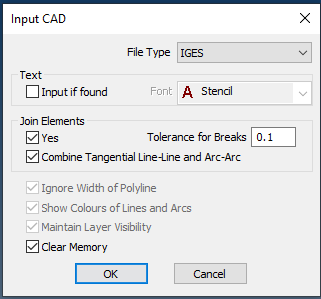# IGES export settings?

I do have a quite nasty situation on exporting some curves as IGES using the latest RhinoWIP.

For some reason I can’t get this curves to be as a single SPLINE in Alphacam 2016 excepting one single file exported and I cant find any reason why the other attempts to export the very same curve it is resulting in segmented splines.

How can I find the settings or configuration that was used to export as IGES?

All the IGES files from the ZIP archive are various attempts to export the same geometry without success. Any of them gets imported in Alphacam as exploded spline in different segment parts.

iges_export.3dm (32.0 KB)

iges_export_good.igs (4.1 KB)

iges.zip (18.5 KB)This is the analysis of your curve:

``````curve

ID: ae2970ef-1c3c-432c-ae23-016e58905166 (2)
Object name: Cut
Layer name: contour
Render Material:
source = from layer
index = -1

Geometry:
Valid curve.
Open polycurve with 6 curve segments.
Segment 1:
Open NURBS curve
start = (501.99,336.126,-34.1405)
end = (497.621,197.068,-48.9185)
degree = 2
control points: non-rational, count=3
knots: uniform (delta=139.127), domain = -139.049 to 0.0780947
clamped at start and end
Segment 2:
Elliptical arc
focal distance = 3.22172
foci = (488.344,197.359,-46.507), (482.11,197.555,-44.8866)
center = (485.227,197.457,-45.6968)
normal = (-0.251343,0.00789689,-0.967866)
start = (497.621,197.068,-48.9185)
end = (485.227,185.057,-45.798)
degree = 2
control points: rational, count=3
knots: uniform (delta=19.0884), domain = 0.0780947 to 19.1665
clamped at start and end
Segment 3:
Open NURBS curve
start = (485.227,185.057,-45.798)
end = (299.998,185.057,-20.4162)
degree = 2
control points: non-rational, count=3
knots: uniform (delta=213.753), domain = 19.1665 to 232.919
clamped at start and end
Segment 4:
Open NURBS curve
start = (299.998,185.057,-20.4162)
end = (114.768,185.057,-45.798)
degree = 2
control points: non-rational, count=3
knots: uniform (delta=213.753), domain = 232.919 to 446.672
clamped at start and end
Segment 5:
Elliptical arc
focal distance = 3.22172
foci = (111.652,197.359,-46.507), (117.885,197.555,-44.8866)
center = (114.768,197.457,-45.6968)
normal = (-0.251343,-0.00789689,0.967866)
start = (114.768,185.057,-45.798)
end = (102.375,197.068,-48.9185)
degree = 2
control points: rational, count=3
knots: uniform (delta=19.0884), domain = 446.672 to 465.76
clamped at start and end
Segment 6:
Open NURBS curve
start = (102.375,197.068,-48.9185)
end = (98.0055,336.126,-34.1405)
degree = 2
control points: non-rational, count=3
knots: uniform (delta=139.127), domain = 465.76 to 604.887
clamped at start and end
``````

So it’s a degree 2 polycurve made up of 6 segments. Then I tested the continuity between the segments and the 4 middle curves are not tangent - by almost 1 degree in the middle and by around 0.3 degrees at the ends. So I guess AlphaCAM might have a problem making it into one continuous curve.

But the very SAME geometry I can’t get to be exported-imported again as one spline. The geometry from the example that I provided it is importing perfectly using the first time IGES exported file. After that, no matter what I do I can’t get it to work again as needed!

The geometry from the file ‘iges_export_good.igs’ it is the same with the Rhino file. The picture with the yellow arrow it is shows only one spline. Any other time I do try to export again the very same geometry I can’t get-it to work as needed, I get only six splines instead of one.

I re-imported the iges_export_good.igs into the iges_export.3dm Rhino file in the WIP and it still shows as a polycurve composed of 6 segments, not a single spline. As a matter of fact, it selects with SelDup and the original.